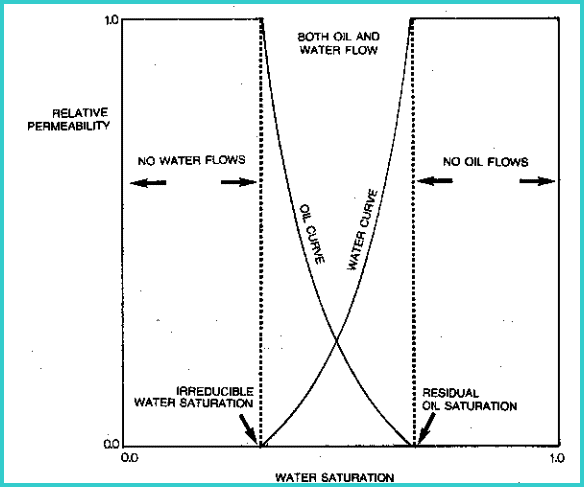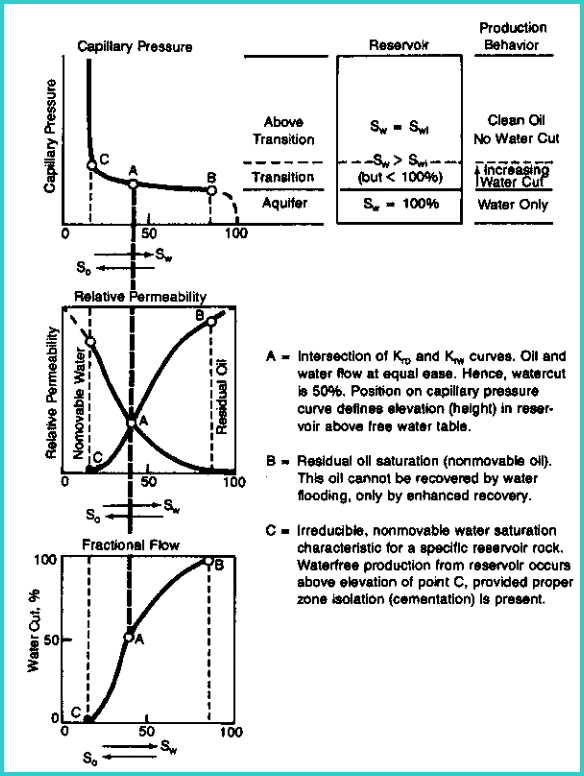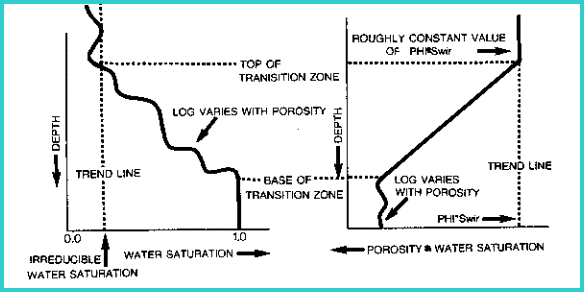PERMEABILITY BASICS
Most quantitative petrophysical log  analysis is aimed at defining shale content, porosity, and water saturation. These terms define the oil or gas in place in the reservoir at initial conditions. What we would really like to know is: "Is the well any good?" That is, will it produce anything, and if so, how much per day. To know this, we must determine values for permeability and productivity.

Permeability, also known as hydraulic conductivity, refers to the ease with which fluids flow through any substance. It is not sufficient to have oil or gas in a formation; the hydrocarbons must be able to flow from the reservoir into the well bore in order to be recovered at the surface. Absolute permeability is a physical characteristic of the rock. Permeability of a rock for oil, gas, or water is a function of the absolute permeability and the viscosity of the fluid.

Productivity describes the flow rate of oil or gas into the well bore. These two terms are obviously related. This Chapter explains how to determine these values from open hole log data.

Reservoir volume is a term used, with other adjectives, to describe the volume of hydrocarbon in the reservoir. It is also called the oil in place or gas in place. The phrases, reserves or recoverable reserves, refer to the amount of hydrocarbon in place that can actually be produced under the existing (or a proposed) recovery mechanism.

Recoverable reserves and productivity define, along with product prices and production costs, whether or not a well will make money; that is, "Is the well any good?"

Permeability is measured by flowing fluids through the rock under known conditions. This can be done on rock samples in the laboratory, or by flowing a well (in -situ measurement). It depends on the size and shape of the pores and pore throats, the properties of the fluids, the pressure exerted on the fluid, and the amount of the fluid flow.Darcy's fluid flow equation
Darcy's fluid flow equation relates these properties to permeability.

For linear horizontal flow:
1: Q = 1.127 * A * (K / MU) * (P1 - P2) / L

Where:
Q = quantity of fluid (bbl/day)
A = area fluid flows through (sq feet)
K = permeability (Darcies)
MU = viscosity of fluid (centipoise)
P1 - P2 = pressure differential (psi)
L = length of flow path (feet)

For non-horizontal flow (eg. up-dip in a reservoir) a gravity term must be included.

More importantly, fluid flow from a reservoir into a wellbore is not linear but radial, so the equation becomes:
2: Q = 3.07 * H * (K / MU) * (Pr - Pb) / log(Rr/Rb)

Where:
Q = quantity of fluid (bbl/day)
H = thickness of reservoir that fluid flows through (feet)
K = permeability (Darcies)
MU = viscosity of fluid (centipoise)
Pr - Pb = pressure differential from reservoir to wellbore(psi)
Rr = radius of reservoir = length of flow path (feet)
Rb = radius of wellbore (feet)

The unit used in measuring permeability is the Darcy. Permeabilities normally encountered in reservoir rocks range from less than one millidarcy in low porosity sandstones, to about fifty Darcies in fractured rock. In tight gas and shale gas reservoirs, natural matrix permeability may be as low as a few microdarcies.

Although there are some general trends of increasing permeability with increasing porosity, these do not necessarily hold for any given situation. If the sand grains are large, then the pore throat diameters are large and the permeability is high. If the size of the sand grains is reduced by a factor of l00, the permeability is considerably smaller, but the porosity will be the same. Smaller pores mean larger surface areas around them, and therefore more resistance to flow (lower permeability). Various authors have proposed equations that account for these effects:
Slichner:               K = 10.2 * D^2 / CK1
Terzaghi:              K = CK2 * D^2 * ((PHIe - 0.13) / (1 - PHIe)^0.33)^2
Uren:                    K = CK3 * D^2 * PHIe^3.31
Kozeny                 K = CK4 * PHIe^3 / ((1 - PHIe^2) / (Sv^2)
Kozeny-Carmen   K = CK5 * PHIe^2 * Rp^2 * Cpur

Where:
CK1 = 10 to 100 depending on porosity and grain packing
CK2 = constant to be determined by calibration
CK3 = constant to be determined by calibration
CK4 = 0.20
CK5 = 0.04444
Cpur = 0.216
D = average grain diameter (cm)
K = permeability (Darcy)
PHIe = porosity ((fractional)
Rp = pore throat radius (microns)
Sv = specific surface area of grains (sq cm)

Since D, Sv. and Rp are not easily measured, these are not very practical equations for use in log analysis. However, they do resemble the form of practical equations presented later in this Chapter. The practical equations give permeability as a function of effective porosity and irreducible water saturation, with some tune-ups for shale volume or pore geometry.PORE THROAT RADIUS
The Kozeny-Carmen equation can be rearranged to calculate pore throat radius (Rp), which helps us to understand pore geometry variations within a reservoir.
1: Rp = (K / (44.44 * PHIe^2))^0.5

Dr Zoltan Barlai has proposed some empirical equations that may also be useful:
2: Rp = 1 / (C * (Vclay + Vsilt)*D + E)
3:      3: Rp = 1 / (G * (Vsh / PHIe^2)*H + J)
4: Rp = Rpmax * (1 - Vsh)^2
Where:
C, D, E, G, H, J = regression coefficients
K = absolute permeability (mS)
M = cementation esponent (unitless)
PHIe = effective porosity (fractional)
Rp = pore throat radius (microns)
Rpmax = representative or maximum pore throat radius in a clean sand (micronss)Definitions of permeability
Permeability measured with only one fluid in the pores is equal to the absolute permeability, because Darcy's equation accounts for the viscosity and pressures involved in the measurement process. The measurement is often made using air as the fluid, but brine is also used. There is a small correction, called the Klinkenberg correction, to reduce air permeability to a liquid permeability.

The absolute permeability is abbreviated as K, Ka, or Kair.

Effective permeability refers to permeability when one fluid flows in the presence of a second fluid in a pore system, for example oil flowing and irreducible water not flowing. Effective permeability is less than absolute permeability because the presence of a second fluid reduces the size of holes available for flow of the first fluid. If no fluid flows, the effective permeability of the rock to that fluid is zero.

Relative permeability is the ratio of effective permeability of a specific fluid to absolute permeability. Graphs of relative permeability curves reflect the capacity of the rock to produce fluids by showing the permeability of those fluids as a function of saturation.Typical relative permeability curves for oil and waterRelative permeability and capillary pressure curve compared

The amount of fluid flowing is not a direct result of the relative permeability, as different fluids have different viscosities. For example, if gas and oil have equal relative permeabilities, more gas than oil will flow within the rock because of the dramatic difference in viscosity.

Most core analysis reports provide three permeability measurements labeled Kmax, K90, and Kvert. Kmax is the permeability in the horizontal direction through the core with the highest permeability. This direction is determined by measuring the pressure drop across the core and then rotating the core until the minimum pressure drop is located. This permeability is sometimes labeled Khor, Khoriz, or Kh.

After rotating the core 90 degrees from the direction of Kmax, K90 is measured. K90 must be less than or equal to Kmax.

Kvert is then measured by flowing fluid through the vertical direction on the core. Kvert is often less than Kmax in sandstones and shaly sandstones. It may be higher than Kmax if semi-vertical fractures exist, as in many carbonate reservoirs.

The permeability derived from log analysis is the absolute permeability, if it is calibrated to the absolute permeability from core data. Usually, log results are calibrated to the maximum permeability from core (Kmax). Effective permeability can usually be derived from absolute permeability, using empirical relationships.

The irregular, narrow connections between pores are called capillaries. They can be likened to thin tubes connecting any two points in the reservoir. Capillary pressure is the phenomenon by which water or any wetting liquid is drawn up into a vertical capillary. The smaller the capillary, the higher the liquid rises.

Due to the variety of capillary diameters, the water saturation within a rock varies above the hydrocarbon-water contact. The water saturation caused by capillary pressure in the hydrocarbon zone is called the irreducible water saturation. A zone at irreducible water saturation will not produce water. A zone that is very wet AND at its irreducible water saturation will not flow water. A zone could have such poor permeability that an apparently wet zone can occur anywhere in an oil or gas column. This problem is solved by calling the apparently wet zone "tight", or impermeable", or "non-pay". It is not a “water zone”.

Between the water and hydrocarbon zones, is a layer of rock filled with both water and oil, with the water at a saturation higher than the irreducible saturation. It is considered the region in which both water and oil (or gas) will flow. It is termed the transition zone. This should not be confused with the same term used to describe the invasion of mud filtrate into the formation.

The more small capillaries there are, the higher the water saturation will be. Also the transition from irreducible water saturation to 100% water throughout the transition zone will be longer. The fraction of water flowing with the oil (or gas) is referred to as the water cut.

These definitions suggest that it is important to know the top depth and base of the transition zone, so that a well can be produced from above the top of the transition zone to minimize water production. This may not be obvious from specific values of water saturation, because the porosity is seldom constant, but it may be more apparent by observing the porosity times water saturation product (PHIxSW) through the transition zone. Compare the illustration on the right side of the illustration below with that on the left.Identifying transition zone from PHI*SW productPermeability of Fractures
The permeability of fractures is a function of the width (aperature) of the fracture. A rough relationship, proposed by Dr Zoltan Barlai, for permeability versus width (in imillimeters) of a fracture is:
10: PHIf = 0.001 * Wf * Df * KF1
11: Kfrac = 833 * 10^11 * PHIfrac^3 / (Df^2 * KF1^2)
12: Kfrac = 833 * 10^5 * PHIfrac * Wf^2
13: Kfrac = 833 * 10^2 * Wf^3 * Df * KF1

Where:
KF1 = number of main fracture directions
= 1 for sub-horizontal or sub-vertical
= 2 for orthogonal sub-vertical
= 3 for chaotic or brecciated
PHIfrac = fracture porosity (fractional)
Df = fracture frequency (fractures per meter)
Wf = fracture aperture (millimeters)
Kfrac = fracture permeability (millidarcies)

Note: Equations 11, 12, and 13 give identical results.

Therefore, a fracture 1 millimeter thick has a permeability of 83 darcies (or 83,000 millidarcies).

Page Views ---- Since 01 Jan 2015
Copyright 2023 by Accessible Petrophysics Ltd.
CPH Logo, "CPH", "CPH Gold Member", "CPH Platinum Member", "Crain's Rules", "Meta/Log", "Computer-Ready-Math", "Petro/Fusion Scripts" are Trademarks of the Author